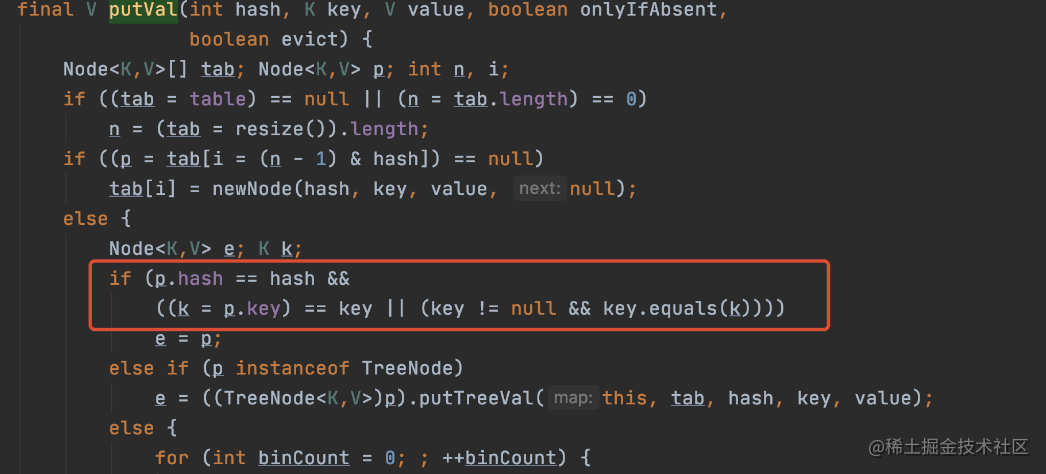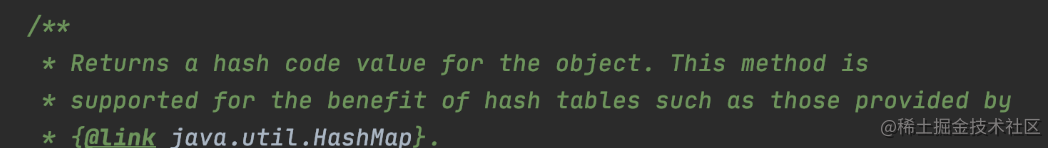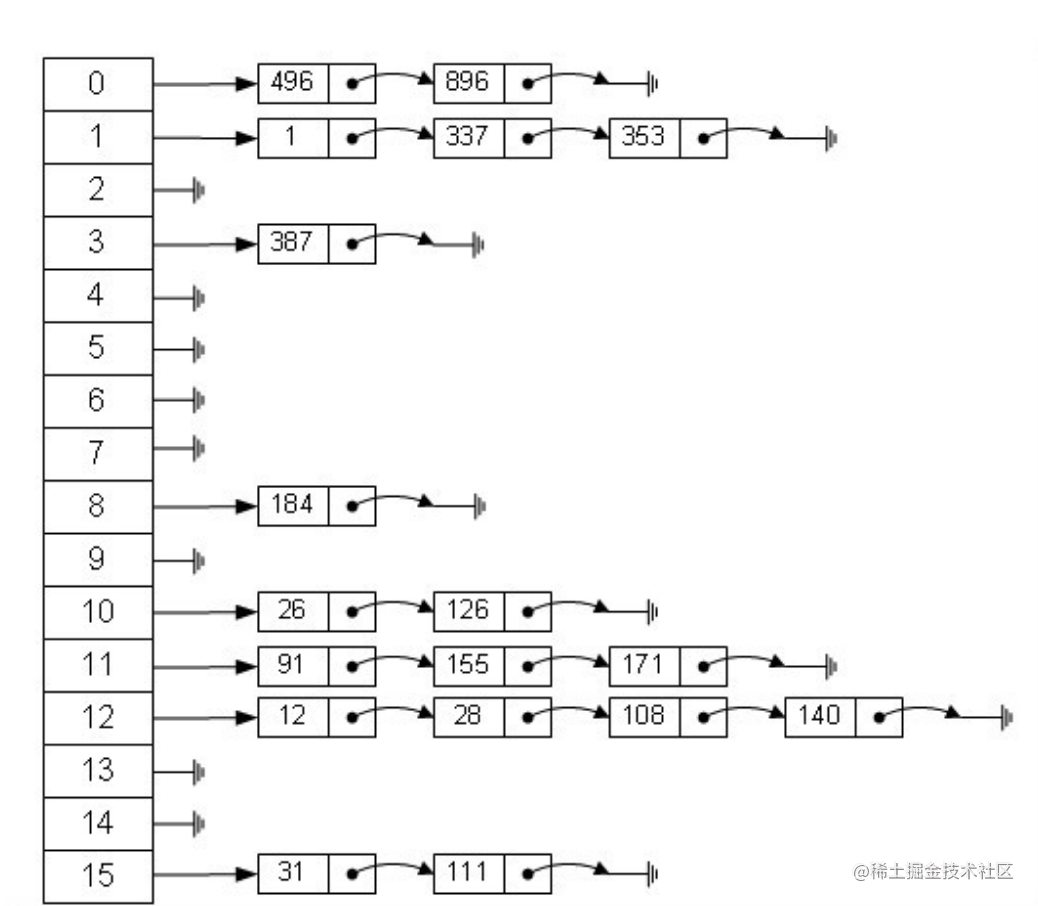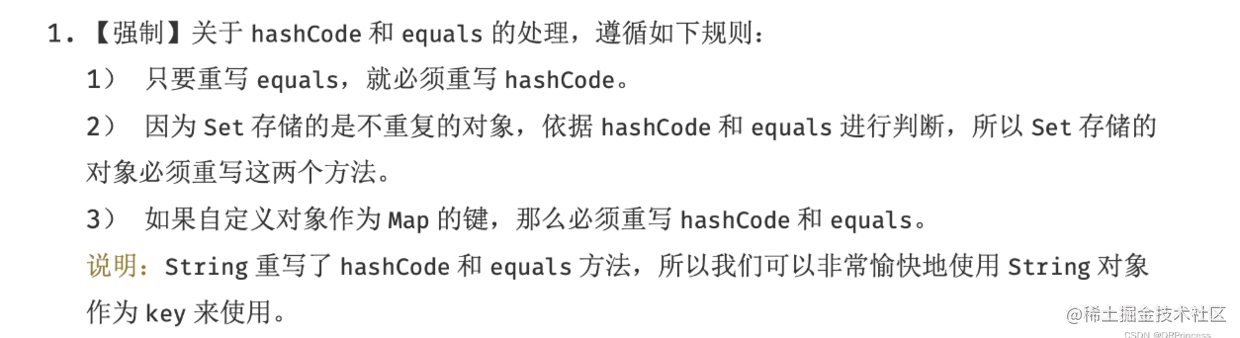# 面試全經過&題目

```java public class Test { class Item{

``````    private int code = 1;

public Item(int code){
this.code =  code;
}

override public int hashCode() {
return code;
}
}

public static void main(String[] args) {
Set<Item> set = new HashSet<>();

System.out.println(set.size());

}
``````

}

```

• 面試官： 這有一道代碼題，你看下，你覺得最終會輸出什麼呢？
• 我：2
• 面試官：為什麼？談談原因？
• 我：因為重寫了hash,HashSet 內部會認為傳遞同樣的code是同一個對象，所以第一個`new Item(0)`和第二個`new Item(0)` 會被認為是同一個對象，然後後者會覆蓋前者，所以是2

• 面試官：實際上這段代碼是不能運行的，現在需要你修改正確，並輸出結果

• 我：編寫代碼中....(Item 改成了 static,override 關鍵字修改為 @Override註釋)
• 我：改完了，運行一看，發現是 3，你説尷尬不尷尬.....
• 面試官：你覺得為啥和你之前設想的不一樣
• 我：應該是還需要實現equals方法
• 面試官：為什麼呢？
• 我：...

# 問題解惑

2. hashCode() 和 equals() 的理解

``````//固定的value值
private static final Object PRESENT = new Object();
``````

``````//添加方法，實際上，將數據作為key存儲到  HashMap 中
return map.put(e, PRESENT)==null;
}
``````

} ```看下分支條件

```if (p.hash == hash && ((k = p.key) == key || (key != null && key.equals(k))))```

• p.hash == hash

```//put 的時候進行hash() public V put(K key, V value) { return putVal(hash(key), key, value, false, true); } //hash算法的實現 static final int hash(Object key) { int h; return (key == null) ? 0 : (h = key.hashCode()) ^ (h >>> 16); }```

• (k = p.key) == key || (key != null && key.equals(k))

## hashCode() 和 equals() 的理解

### 首先它們是什麼？

```

``````public boolean equals(Object obj) {
return (this == obj);
}

@HotSpotIntrinsicCandidate
public native int hashCode();
``````

```

equals() ：內部使用了 “==”。判斷是否是同個對象，如果是值類型，判斷內容是否一致，如果是引用類型，則是引用地址是否相等。 hashCode()：一個本地方法，主要看native 中的實現。

1. 隨機數
2. 內存地址做移位再和一個隨機數做異或
3. 固定值1
4. 自增序列的當前值
5. 內存地址
6. 當前線程有關的一個隨機數+三個確定值，運用xorshift隨機數算法得到的一個隨機數。

### 然後看為什麼？兩個方法的設計目的• equals() 的默認實現是用的內存地址，但是也有很多類改寫了，例如 String 就修改了。
• equals() 允許重寫後，邏輯可能會變得複雜，所以就性能上來説，大概率沒有 hashCode() 的性能高。

```if (p.hash == hash && ((k = p.key) == key || (key != null && key.equals(k))))```

### 然後怎麼用？重寫 equals() 和 hashCode() 的注意事項

``` // String.java public boolean equals(Object anObject) { //內存地址相等，肯定是同個對象 if (this == anObject) { return true; } //否則逐個判斷字符是否一致 if (anObject instanceof String) { String aString = (String)anObject; if (!COMPACT_STRINGS || this.coder == aString.coder) { return StringLatin1.equals(value, aString.value); } } return false; }

``````public int hashCode() {
int h = hash;
if (h == 0 && !hashIsZero) {
h = isLatin1() ? StringLatin1.hashCode(value)
: StringUTF16.hashCode(value);
if (h == 0) {
hashIsZero = true;
} else {
hash = h;
}
}
return h;
}
``````

//StringLatin1.java

``````//哈希算法的真正實現
public static int hashCode(byte[] value) {
int h = 0;
for (byte v : value) {
h = 31 * h + (v & 0xff);
}
return h;
}

//逐個判斷字符是否一致
public static boolean equals(byte[] value, byte[] other) {
if (value.length == other.length) {
for (int i = 0; i < value.length; i++) {
if (value[i] != other[i]) {
return false;
}
}
return true;
}
return false;
}
``````

```

equals()重寫的時候需要滿足以下規則：

• 內存地址相同，一定返回true (使用“==”操作符檢查)
• 類型不一致，一定返回false(使用instanceof 操作符檢查)
• 類型轉換後，判斷類中每個關鍵屬性，符合預設，返回 true , 否則 false

hashCode()重寫的時候需要滿足以下規則：

• 保證同一個對象，多次調用 Hashcode 是一致的。
• equals()相等，hashCode()肯定相等# 總結

1. 不受重寫 hashCode() 的干擾，給出正確的列表長度答案
2. 説出對 hashCode() 和 equals() 的理解

# 參考資料

Effective Java 中文版

Java Object.hashCode()返回的是對象內存地址？